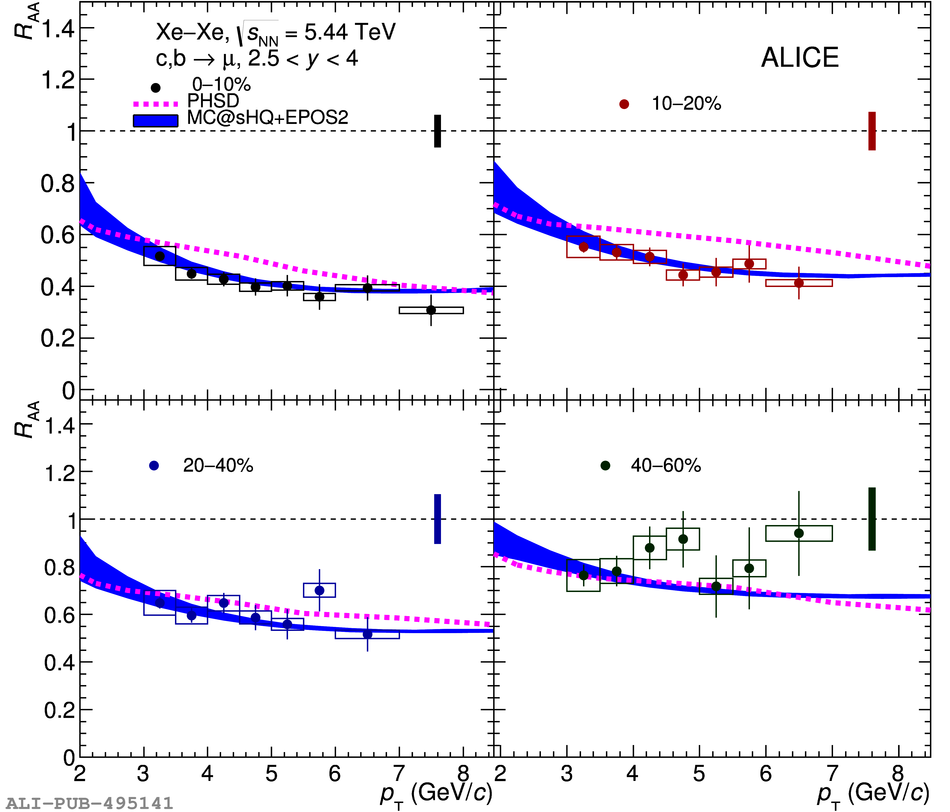Figure 2

 Nuclear modification factor $\RAA$ of muons from heavy-flavour hadron decays at forward rapidity as a function of $\pT$ in Xe--Xe collisions at$\sqrt{s_{\rm NN}}$ = 5.44 TeV for various centrality intervals mentioned in the figure. Statistical uncertainties (vertical bars) and systematic uncertainties (open boxes) are shown. The filled boxes at $\RAA$ = 1 represent the normalisation uncertainty. Comparisons with the PHSD~ and MC@sHQ+EPOS2~ models are presented.